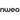# Subtracting fractions: 3 crucial steps you absolutely need

All Posts
Subtracting is the opposite of addition, something your students have learned well before exploring the world of fractions. Subtracting fractions is a little bit more complicated than regular deduction, but there is a teaching strategy that makes the process as easy as one, two, three!In this article, we’ll explore several topics, including:Check out these related articles for more information on fractions:

## What are fractions?

Fractions represent parts of a whole. When a whole number is broken up into different parts, a fraction shows how much of it you have.For example, the fraction ¾ indicates there are three of the four parts needed to make a whole number.Fractions are made up of two parts, the numerator and denominator, which are separated by a horizontal line.The denominator is the bottom number of a fraction and represents how many parts the whole number has been split into. The fraction ¾ has been split into four parts. https://www.instagram.com/p/BtuP1F5lKek/ The numerator is the top number of a fraction. It represents how many parts of the whole number you have. So, the fraction ¾ means you have three out of four parts.

## What is subtraction?

Subtraction involves taking the value of one number away from another. Subtraction is represented with a dash (-), or a minus symbol.The number that appears before the minus symbol is the value that’s being subtracted from. The number that appears after the minus symbol is what the first number is being subtracted by.Let’s look at an example:

`8 - 3 = ?`

In this equation, three is being taken away from eight. Counting down three numbers from eight leaves the solution at five.

`8 - 3 = 5`

## 3 Easy steps for subtracting fractions

Subtracting fractions can be done in three easy steps:
• Make the denominators the same
• Subtract the numerators, putting the answer over the same denominator
• Simplify if possible (and if required)
Like most equations involving fractions, students will have to do a little extra work before finding the solution.

### 1. Make the denominators the same

To subtract fractions, the denominators in the equation need to be the same. When subtracting fractions with unlike denominators, teach your students to first find the lowest common denominator.Think of a fraction as part of a circle. Changing the denominators doesn’t change the amount of the circle students have, but rather the number of parts it’s being divided into.Once students have equal denominators, or parts, it becomes much easier to see how they can subtract the fractions.Consider this problem: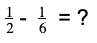In this example, students need to take away a sixth from a half. The first step is to find a common denominator, so these fractions are split into the same number of parts. To find the lowest common denominator, multiply the denominators together. Remember, whatever is done to the denominator needs to be done to the numerator as well.½ is multiplied by six, while ⅙ is multiplied by two.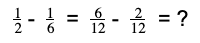Now students can see ½ is the same as saying ⁶⁄₁₂ and ⅙ is the same as saying ²⁄₁₂.### 2. Subtract the numerators, putting the answer over the same denominator

Now that the denominators are the same, tell your students to put both numerators over one denominator and simply subtract them from each other. This is to see how many parts of twelve are being subtracted from the original fraction.In the example equation, students are taking two parts of twelve away from six parts of twelve, leaving four parts of twelve.

### 3. Simplify if possible (and if required)

Simplifying means reducing a fraction to its lowest possible multiple. Remember: what’s done to the denominator is also done to the numerator.Make sure you tell students if it’s required for them to simplify solutions. Some teachers don’t make it necessary to simplify solutions, while others don’t consider a question complete until the solution has been put to its simplest form. https://www.instagram.com/p/BuUAVYZDiBP/ When you teach fractions, make sure your students understand what your expectations are.To simplify a fraction, figure out its lowest common multiple. One quick way to do this is by seeing if the denominator can be divided by the numerator. In the example, four does indeed go into twelve. To simplify this equation, divide both the numerator and the denominator by four.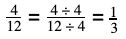After completing all the calculations, students are left with one third.

## Different types of fractions

Your students will encounter several different types of fractions throughout their studies, but the process for these problems remains almost entirely the same.

### Subtracting fractions with like denominators

Fraction equations with like denominators already have the first step of the three-step process done for them.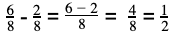Tell students they can jump straight to the second step and put both numerators over a single denominator.

### Subtracting improper fractions

An improper fraction is when the numerator is greater than the denominator. This means there is more than one whole number within the fraction. Tell your students to imagine it as a complete circle along with an incomplete circle.Luckily, there are no changes in how these types of equations are solved.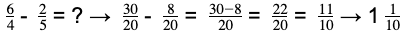The solution can either be left as an improper fraction or changed into a mixed fraction.

### Subtracting fractions with mixed numbers

A mixed number, or mixed fraction, is made of both a whole number and a fraction. To solve equations with mixed numbers, teach your students to change it into an improper fraction first.To do this, multiply the whole number by the denominator and then add it to the numerator.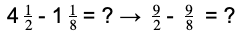Once you’ve shown students how to create an improper fraction, they can continue with the three-step process for subtracting fractions.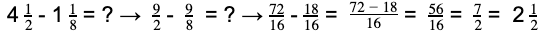### Subtracting fractions with whole numbers

Equations with whole numbers need to be changed into fractions. To turn a whole number into a fraction, make the whole number the numerator and then put it over a denominator of one.For example:Students can then solve the equation with the three-step process.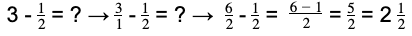## How Prodigy can help teach subtracting fractions

Are you looking for the perfect activity to supplement your lessons? Look no further than Prodigy!Our game enhances the student learning experience with engaging content, personalized lessons, and fun activities that will make them want to keep playing after class!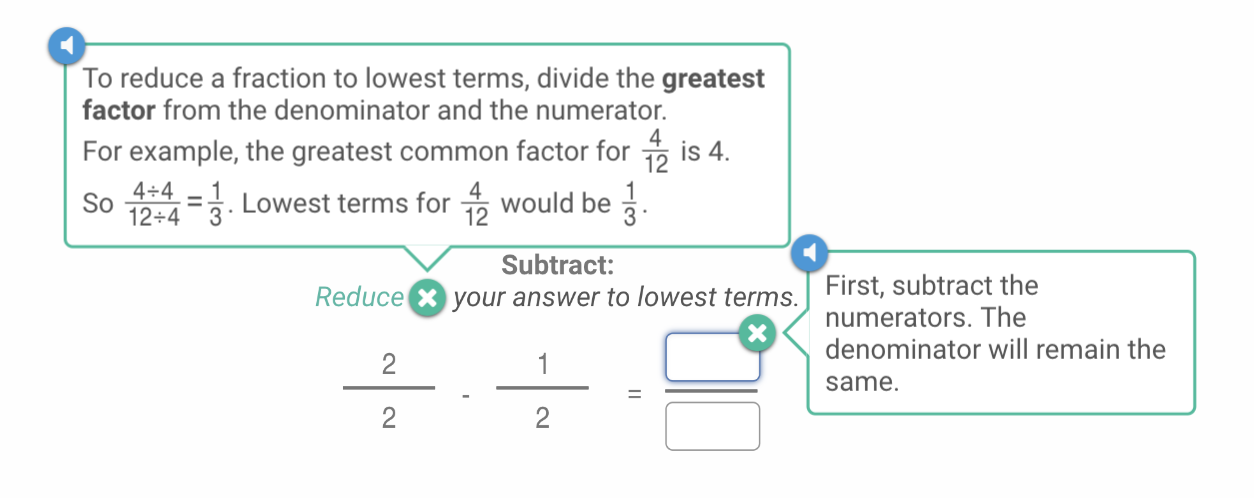Prodigy can help you teach all kinds of math lessons, track how your students are absorbing content in real-time, and assign specific questions to help your class prepare for upcoming tests. Our software is free for all educators, and will remain free — forever!The teacher dashboard gives you access to detailed statistics about your class. Marking is done instantly, so you can see where students are excelling and where they’re struggling. With these up-to-date stats, you can quickly update various students’ gaming experiences to help them learn the subjects they struggle with.Prodigy is curriculum-aligned, so you can feel comfortable using it in tandem with your lessons. Use teacher tools to create assignments, practice tests, and map lesson plans. Check out Prodigy today to see if it’s right for your classroom — for free!

## Adding and subtracting fractions worksheets

Worksheets are a great teaching tool to see how students are comprehending lessons. The only drawback is it can take a long time to mark worksheets from an entire class and give proper feedback to students.Here are some places where you can find worksheets (with answers) for your class:

### K5 Learning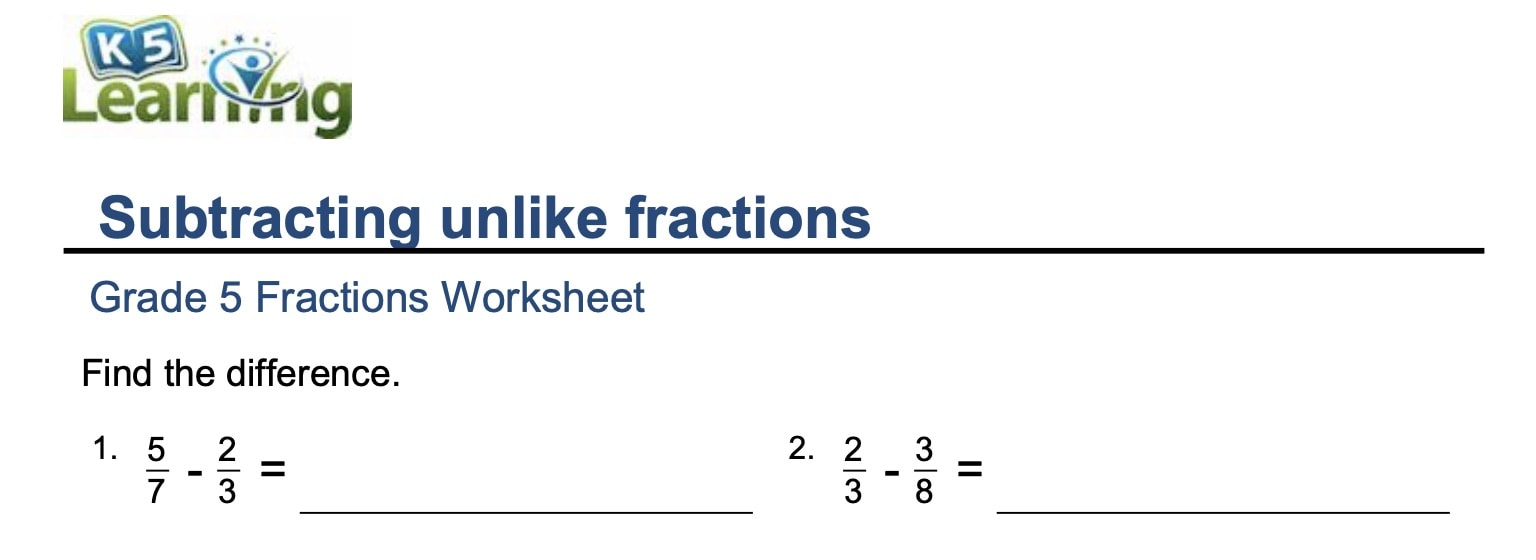K5 Learning offers a wide variety of worksheets for different areas of math. The math worksheets only go up to fifth grade, but they’re in-depth and cover many areas of the curriculum. All are free to use and come equipped with an answer key.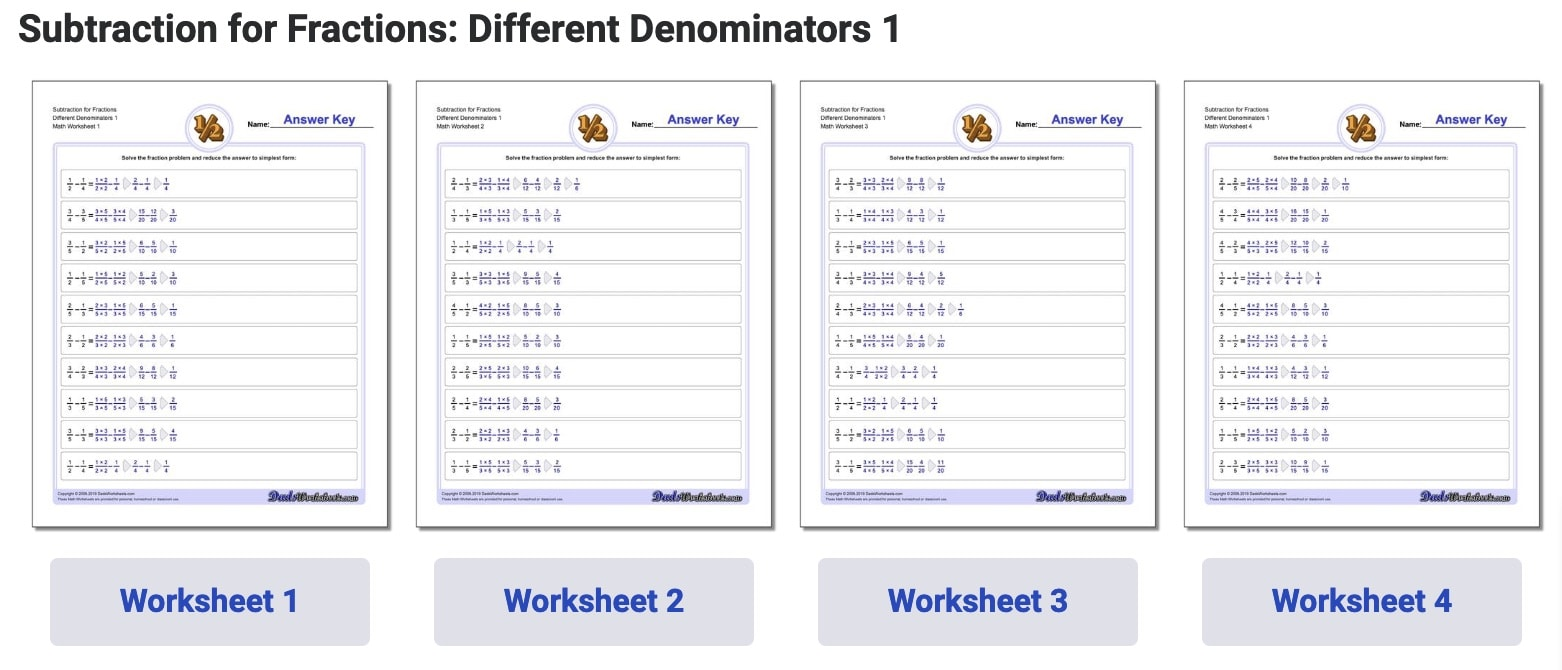At DadsWorksheets.com you can find worksheets for pretty much anything you’re looking for. The worksheets can be downloaded or printed from the web page and come with an answer key.

### Math Drills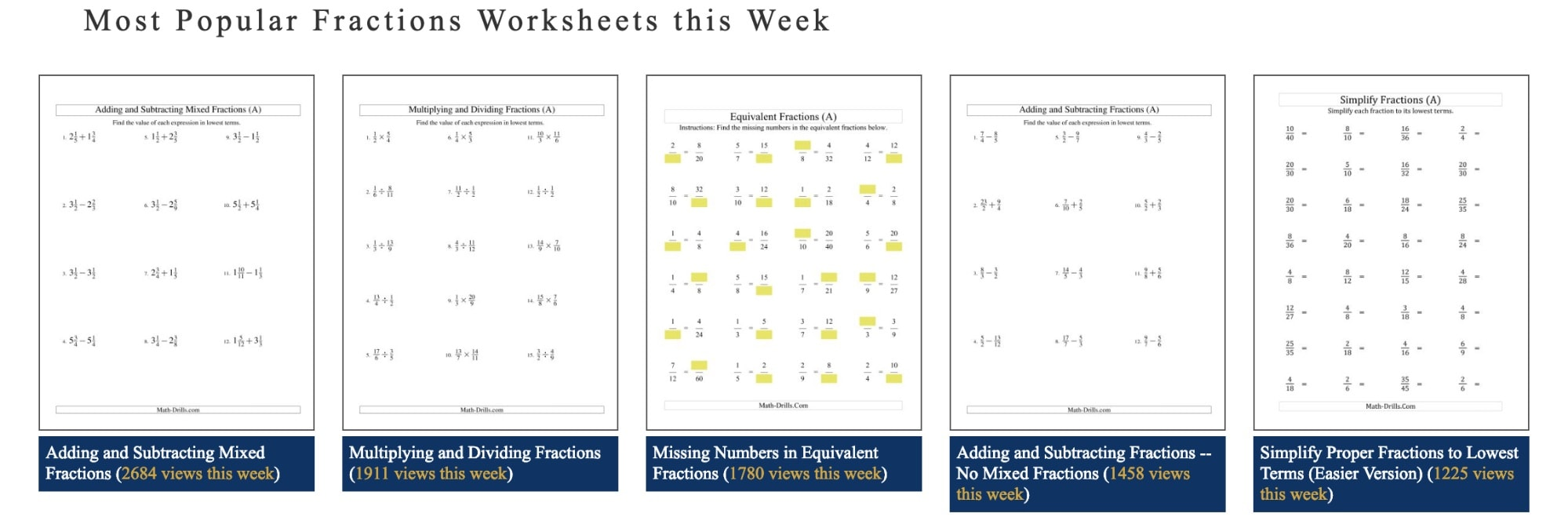Help students master math skills with practice worksheets from Math Drills. These worksheets come with an answer key and cover several subjects you may teach in class. Worksheets are free and can be downloaded and/or printed right from their website.

## Conclusion: subtracting fractions

The process for subtracting fractions is quite similar to adding fractions. Teach your students they can solve these types of equations in three steps:
1. Make the denominators the same
2. Subtract the numerators, putting them over one denominator
3. Simplify if possible
Visualizing fractions as partially filled shapes, like a circle, can help students understand what they’re trying to accomplish. Think of subtracting fractions like taking a slice of pie away from the rest of the pie.With this strategy at their disposal, students will be ready to tackle fractions in class, and as they appear throughout their lives.
Create or log into your teacher account on Prodigy — a free, game-based learning platform for math that's easy to use for educators and students alike. Aligned with curricula across the English-speaking world, it's loved by more than 1.5 million teachers and 50 million students.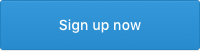•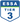•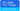•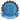•